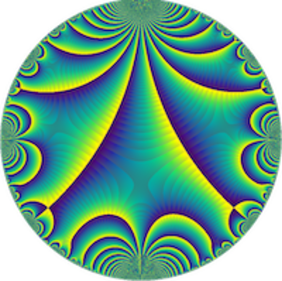# Convolution of periodic multiplicative functions and the divisor problem — Gopal Maiti (Aix-Marseille Université)

Séminaire « Arithmétique »
M2 Kampé de Fériet

I will speak about a joint work with Aymone, Ramaré, and Srivastav where we showed optimal Omega bounds for the partial sums of the convolution of two periodic multiplicative functions with bounded partial sums (except Dirichlet characters). I will also discuss the autocorrelations of the delta function and how this relates to our main theorem.